# kornia.morphology#

kornia.morphology.dilation(tensor, kernel, structuring_element=None, origin=None, border_type='geodesic', border_value=0.0, max_val=1e4, engine='unfold')[source]#

Return the dilated image applying the same kernel in each channel.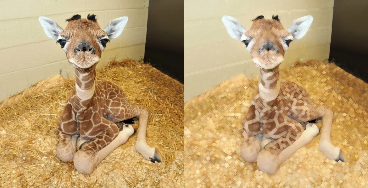The kernel must have 2 dimensions.

Parameters:
• tensor (Tensor) – Image with shape $$(B, C, H, W)$$.

• kernel (Tensor) – Positions of non-infinite elements of a flat structuring element. Non-zero values give the set of neighbors of the center over which the operation is applied. Its shape is $$(k_x, k_y)$$. For full structural elements use torch.ones_like(structural_element).

• structuring_element (, optional) – Structuring element used for the grayscale dilation. It may be a non-flat structuring element. Default: None

• origin (, optional) – Origin of the structuring element. Default: None and uses the center of the structuring element as origin (rounding towards zero).

• border_type (str, optional) – It determines how the image borders are handled, where border_value is the value when border_type is equal to constant. Default: geodesic which ignores the values that are outside the image when applying the operation.

• border_value (float, optional) – Value to fill past edges of input if border_type is constant. Default: 0.0

• max_val (float, optional) – The value of the infinite elements in the kernel. Default: 1e4

• engine (str, optional) – convolution is faster and less memory hungry, and unfold is more stable numerically Default: "unfold"

Return type:

Tensor

Returns:

Dilated image with shape $$(B, C, H, W)$$.

Note

See a working example here.

Example

>>> tensor = torch.rand(1, 3, 5, 5)
>>> kernel = torch.ones(3, 3)
>>> dilated_img = dilation(tensor, kernel)

kornia.morphology.erosion(tensor, kernel, structuring_element=None, origin=None, border_type='geodesic', border_value=0.0, max_val=1e4, engine='unfold')[source]#

Return the eroded image applying the same kernel in each channel.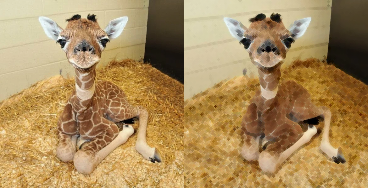The kernel must have 2 dimensions.

Parameters:
• tensor (Tensor) – Image with shape $$(B, C, H, W)$$.

• kernel (Tensor) – Positions of non-infinite elements of a flat structuring element. Non-zero values give the set of neighbors of the center over which the operation is applied. Its shape is $$(k_x, k_y)$$. For full structural elements use torch.ones_like(structural_element).

• structuring_element (torch.Tensor, optional) – Structuring element used for the grayscale dilation. It may be a non-flat structuring element. Default: None

• origin (, optional) – Origin of the structuring element. Default: None and uses the center of the structuring element as origin (rounding towards zero).

• border_type (str, optional) – It determines how the image borders are handled, where border_value is the value when border_type is equal to constant. Default: geodesic which ignores the values that are outside the image when applying the operation.

• border_value (float, optional) – Value to fill past edges of input if border_type is constant. Default: 0.0

• max_val (float, optional) – The value of the infinite elements in the kernel. Default: 1e4

• engine (str, optional) – convolution is faster and less memory hungry, and unfold is more stable numerically Default: "unfold"

Return type:

Tensor

Returns:

Eroded image with shape $$(B, C, H, W)$$.

Note

See a working example here.

Example

>>> tensor = torch.rand(1, 3, 5, 5)
>>> kernel = torch.ones(5, 5)
>>> output = erosion(tensor, kernel)

kornia.morphology.opening(tensor, kernel, structuring_element=None, origin=None, border_type='geodesic', border_value=0.0, max_val=1e4, engine='unfold')[source]#

Return the opened image, (that means, dilation after an erosion) applying the same kernel in each channel.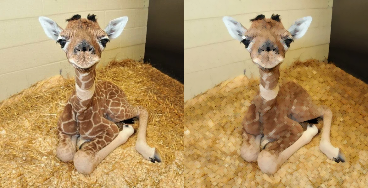The kernel must have 2 dimensions.

Parameters:
• tensor (Tensor) – Image with shape $$(B, C, H, W)$$.

• kernel (Tensor) – Positions of non-infinite elements of a flat structuring element. Non-zero values give the set of neighbors of the center over which the operation is applied. Its shape is $$(k_x, k_y)$$. For full structural elements use torch.ones_like(structural_element).

• structuring_element (, optional) – Structuring element used for the grayscale dilation. It may be a non-flat structuring element. Default: None

• origin (, optional) – Origin of the structuring element. Default: None and uses the center of the structuring element as origin (rounding towards zero).

• border_type (str, optional) – It determines how the image borders are handled, where border_value is the value when border_type is equal to constant. Default: geodesic which ignores the values that are outside the image when applying the operation.

• border_value (float, optional) – Value to fill past edges of input if border_type is constant. Default: 0.0

• max_val (float, optional) – The value of the infinite elements in the kernel. Default: 1e4

• engine (str, optional) – convolution is faster and less memory hungry, and unfold is more stable numerically Default: "unfold"

Returns:

Opened image with shape $$(B, C, H, W)$$.

Return type:

torch.Tensor

Note

See a working example here.

Example

>>> tensor = torch.rand(1, 3, 5, 5)
>>> kernel = torch.ones(3, 3)
>>> opened_img = opening(tensor, kernel)

kornia.morphology.closing(tensor, kernel, structuring_element=None, origin=None, border_type='geodesic', border_value=0.0, max_val=1e4, engine='unfold')[source]#

Return the closed image, (that means, erosion after a dilation) applying the same kernel in each channel.The kernel must have 2 dimensions.

Parameters:
• tensor (Tensor) – Image with shape $$(B, C, H, W)$$.

• kernel (Tensor) – Positions of non-infinite elements of a flat structuring element. Non-zero values give the set of neighbors of the center over which the operation is applied. Its shape is $$(k_x, k_y)$$. For full structural elements use torch.ones_like(structural_element).

• structuring_element (, optional) – Structuring element used for the grayscale dilation. It may be a non-flat structuring element. Default: None

• origin (, optional) – Origin of the structuring element. Default is None and uses the center of the structuring element as origin (rounding towards zero). Default: None

• border_type (str, optional) – It determines how the image borders are handled, where border_value is the value when border_type is equal to constant. Default: geodesic which ignores the values that are outside the image when applying the operation.

• border_value (float, optional) – Value to fill past edges of input if border_type is constant. Default: 0.0

• max_val (float, optional) – The value of the infinite elements in the kernel. Default: 1e4

• engine (str, optional) – convolution is faster and less memory hungry, and unfold is more stable numerically Default: "unfold"

Return type:

Tensor

Returns:

Closed image with shape $$(B, C, H, W)$$.

Note

See a working example here.

Example

>>> tensor = torch.rand(1, 3, 5, 5)
>>> kernel = torch.ones(3, 3)
>>> closed_img = closing(tensor, kernel)

kornia.morphology.gradient(tensor, kernel, structuring_element=None, origin=None, border_type='geodesic', border_value=0.0, max_val=1e4, engine='unfold')[source]#

Return the morphological gradient of an image.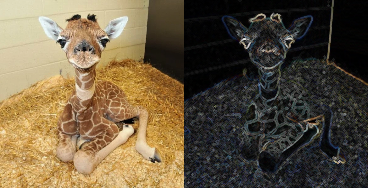That means, (dilation - erosion) applying the same kernel in each channel. The kernel must have 2 dimensions.

Parameters:
• tensor (Tensor) – Image with shape $$(B, C, H, W)$$.

• kernel (Tensor) – Positions of non-infinite elements of a flat structuring element. Non-zero values give the set of neighbors of the center over which the operation is applied. Its shape is $$(k_x, k_y)$$. For full structural elements use torch.ones_like(structural_element).

• structuring_element (, optional) – Structuring element used for the grayscale dilation. It may be a non-flat structuring element. Default: None

• origin (, optional) – Origin of the structuring element. Default is None and uses the center of the structuring element as origin (rounding towards zero). Default: None

• border_type (str, optional) – It determines how the image borders are handled, where border_value is the value when border_type is equal to constant. Default: geodesic which ignores the values that are outside the image when applying the operation.

• border_value (float, optional) – Value to fill past edges of input if border_type is constant. Default: 0.0

• max_val (float, optional) – The value of the infinite elements in the kernel. Default: 1e4

• engine (str, optional) – convolution is faster and less memory hungry, and unfold is more stable numerically Default: "unfold"

Return type:

Tensor

Returns:

Gradient image with shape $$(B, C, H, W)$$.

Note

See a working example here.

Example

>>> tensor = torch.rand(1, 3, 5, 5)
>>> kernel = torch.ones(3, 3)

kornia.morphology.top_hat(tensor, kernel, structuring_element=None, origin=None, border_type='geodesic', border_value=0.0, max_val=1e4, engine='unfold')[source]#

Return the top hat transformation of an image.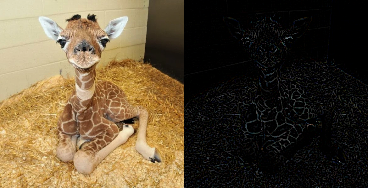That means, (image - opened_image) applying the same kernel in each channel. The kernel must have 2 dimensions.

See opening() for details.

Parameters:
• tensor (Tensor) – Image with shape $$(B, C, H, W)$$.

• kernel (Tensor) – Positions of non-infinite elements of a flat structuring element. Non-zero values give the set of neighbors of the center over which the operation is applied. Its shape is $$(k_x, k_y)$$. For full structural elements use torch.ones_like(structural_element).

• structuring_element (, optional) – Structuring element used for the grayscale dilation. It may be a non-flat structuring element. Default: None

• origin (, optional) – Origin of the structuring element. Default: None and uses the center of the structuring element as origin (rounding towards zero).

• border_type (str, optional) – It determines how the image borders are handled, where border_value is the value when border_type is equal to constant. Default: geodesic which ignores the values that are outside the image when applying the operation.

• border_value (float, optional) – Value to fill past edges of input if border_type is constant. Default: 0.0

• max_val (float, optional) – The value of the infinite elements in the kernel. Default: 1e4

• engine (str, optional) – convolution is faster and less memory hungry, and unfold is more stable numerically Default: "unfold"

Return type:

Tensor

Returns:

Top hat transformed image with shape $$(B, C, H, W)$$.

Note

See a working example here.

Example

>>> tensor = torch.rand(1, 3, 5, 5)
>>> kernel = torch.ones(3, 3)
>>> top_hat_img = top_hat(tensor, kernel)

kornia.morphology.bottom_hat(tensor, kernel, structuring_element=None, origin=None, border_type='geodesic', border_value=0.0, max_val=1e4, engine='unfold')[source]#

Return the bottom hat transformation of an image.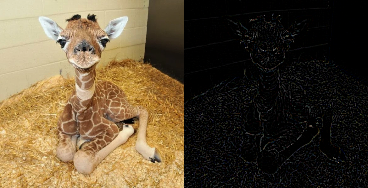That means, (closed_image - image) applying the same kernel in each channel. The kernel must have 2 dimensions.

See closing() for details.

Parameters:
• tensor (Tensor) – Image with shape $$(B, C, H, W)$$.

• kernel (Tensor) – Positions of non-infinite elements of a flat structuring element. Non-zero values give the set of neighbors of the center over which the operation is applied. Its shape is $$(k_x, k_y)$$. For full structural elements use torch.ones_like(structural_element).

• structuring_element (, optional) – Structuring element used for the grayscale dilation. It may be a non-flat structuring element. Default: None

• origin (, optional) – Origin of the structuring element. Default: None and uses the center of the structuring element as origin (rounding towards zero).

• border_type (str, optional) – It determines how the image borders are handled, where border_value is the value when border_type is equal to constant. Default: geodesic which ignores the values that are outside the image when applying the operation.

• border_value (float, optional) – Value to fill past edges of input if border_type is constant. Default: 0.0

• max_val (float, optional) – The value of the infinite elements in the kernel. Default: 1e4

• engine (str, optional) – convolution is faster and less memory hungry, and unfold is more stable numerically Default: "unfold"

Return type:

Tensor

Returns:

Top hat transformed image with shape $$(B, C, H, W)$$.

Note

See a working example here.

Example

>>> tensor = torch.rand(1, 3, 5, 5)
>>> kernel = torch.ones(3, 3)
>>> bottom_hat_img = bottom_hat(tensor, kernel)


## Interactive Demo#

Visit the demo on Hugging Face Spaces.